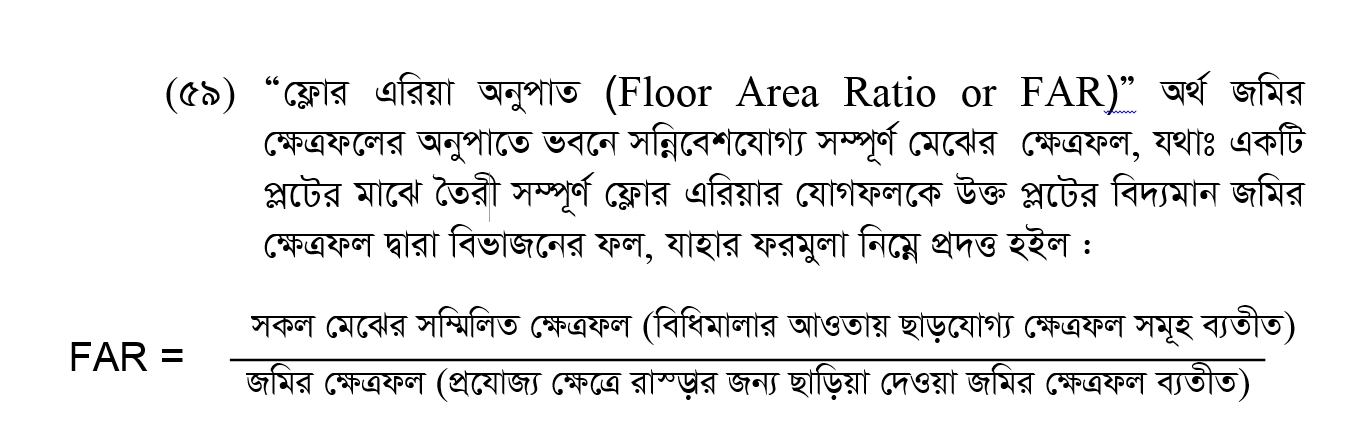# FAR CALCULATION# FAR Calculation of Rajuk

You can get idea of FAR Calculation of your plot by using the following FAR Calculator.

### FAR CalculationClick for LAW GUIDE

Following is a Floor Area Ratio Calculator you can use

#floor_area_ratio_calculator

# Results

• Land area (sft)
• Given land width (ft)